# NCERT Solutions for Class 8 Maths Chapter 2 Linear Equations in One Variable Ex 2.3

NCERT Solutions for Class 8 Maths Chapter 2 Linear Equations in One Variable Ex 2.3 are part of NCERT Solutions for Class 8 Maths. Here we have given NCERT Solutions for Class 8 Maths Chapter 2 Linear Equations in One Variable Ex 2.3.

 Board CBSE Textbook NCERT Class Class 8 Subject Maths Chapter Chapter 2 Chapter Name Linear Equations in One Variable Exercise Ex 2.3 Number of Questions Solved 1 Category NCERT Solutions

## NCERT Solutions for Class 8 Maths Chapter 2 Linear Equations in One Variable Ex 2.3

Question 1.
Solve the following equations and check your results:
1. 3x=2x+18
2. 5t-3=3t-5
3. 5x+9=5+3x
4. 4z+3=6+2z
5. 2x-1=14-x
6. 8x+4=3(x-1)+7
7. $$x=\frac { 4 }{ 5 } \left( x+10 \right)$$
8. $$\frac { 2x }{ 3 } +1=\frac { 7x }{ 15 } +3$$
9. $$2y+\frac { 5 }{ 3 } =\frac { 26 }{ 3 } -y$$
10. $$3m=5m-\frac { 8 }{ 5 }$$
Solution.
1. 3x=2x+18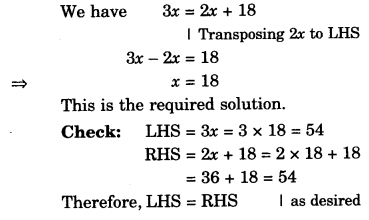2. 5t-3=3t-5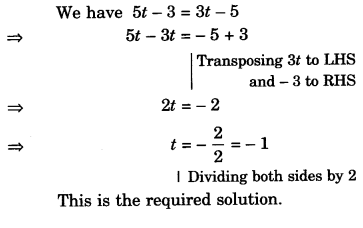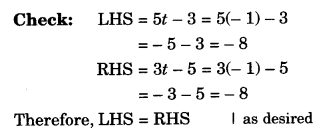3. 5x+9=5+3x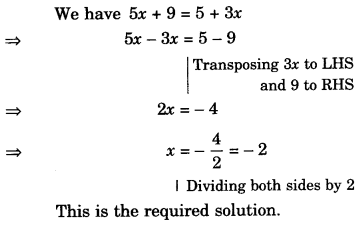4. 4z+3=6+2z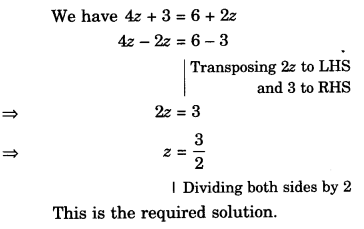5. 2x-1=14-x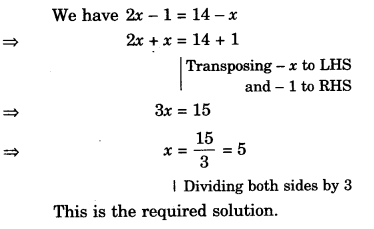6. 8x+4=3(x-1)+7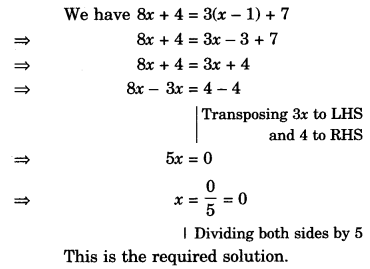7. $$x=\frac { 4 }{ 5 } \left( x+10 \right)$$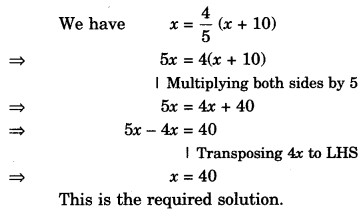8. $$\frac { 2x }{ 3 } +1=\frac { 7x }{ 15 } +3$$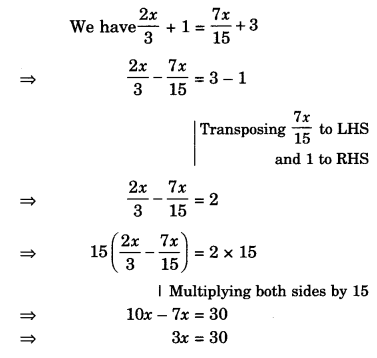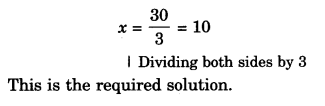9. $$2y+\frac { 5 }{ 3 } =\frac { 26 }{ 3 } -y$$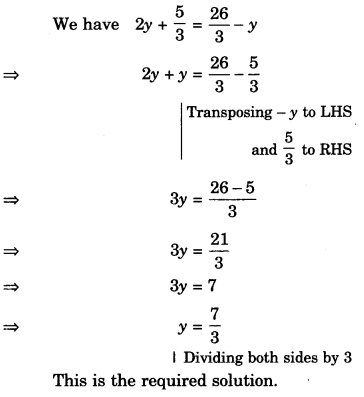10. $$3m=5m-\frac { 8 }{ 5 }$$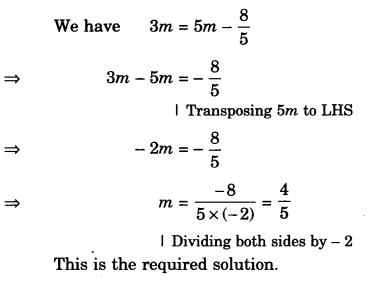We hope the NCERT Solutions for Class 8 Maths Chapter 2 Linear Equations in One Variable Ex 2.3 help you. If you have any query regarding NCERT Solutions for Class 8 Maths Chapter 2 Linear Equations in One Variable Ex 2.3, drop a comment below and we will get back to you at the earliest.